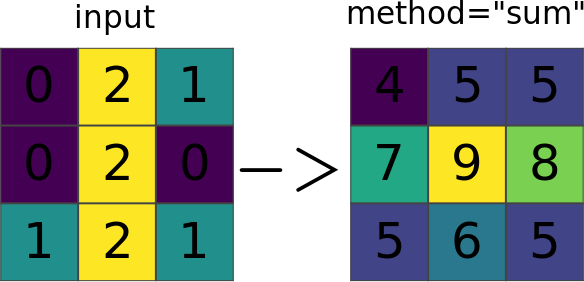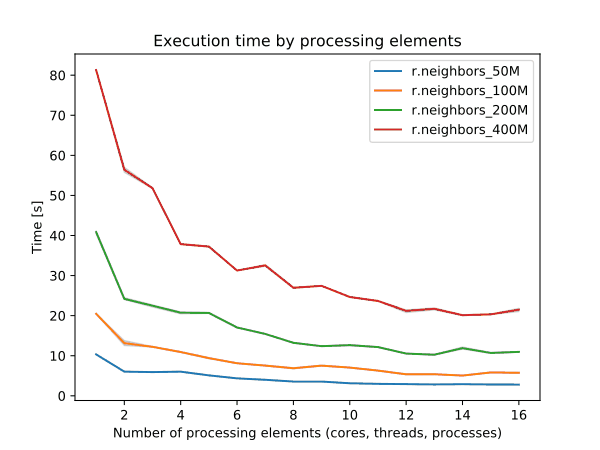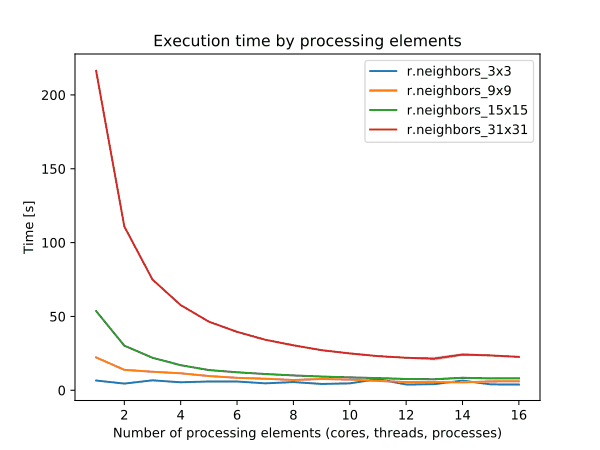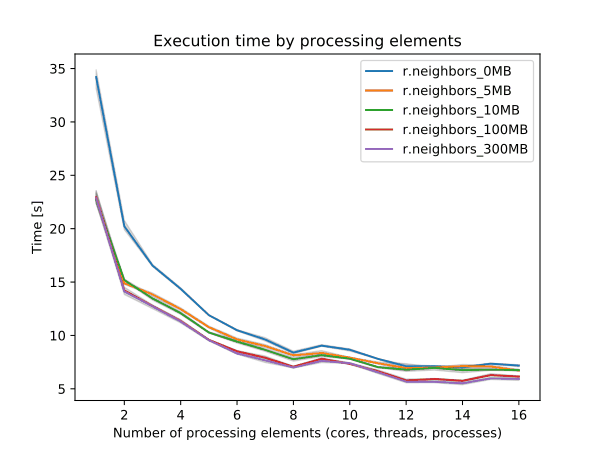## NAME

r.neighbors - Makes each cell category value a function of the category values assigned to the cells around it, and stores new cell values in an output raster map layer.

## KEYWORDS

raster, algebra, statistics, aggregation, neighbor, focal statistics, filter, parallel

## SYNOPSIS

r.neighbors
r.neighbors --help
r.neighbors [-ac] input=name [selection=name] output=name[,name,...] [size=integer] [method=string[,string,...]] [weighting_function=string] [weighting_factor=float] [weight=name] [quantile=float[,float,...]] [title=phrase] [nprocs=integer] [memory=memory in MB] [--overwrite] [--help] [--verbose] [--quiet] [--ui]

### Flags:

-a
Do not align output with the input
-c
Use circular neighborhood
--overwrite
Allow output files to overwrite existing files
--help
Print usage summary
--verbose
Verbose module output
--quiet
Quiet module output
--ui
Force launching GUI dialog

### Parameters:

input=name [required]
Name of input raster map
selection=name
Name of an input raster map to select the cells which should be processed
output=name[,name,...] [required]
Name for output raster map
size=integer
Neighborhood size
Default: 3
method=string[,string,...]
Neighborhood operation
Options: average, median, mode, minimum, maximum, range, stddev, sum, count, variance, diversity, interspersion, quart1, quart3, perc90, quantile
Default: average
weighting_function=string
Weighting function
Options: none, gaussian, exponential, file
Default: none
none: No weighting
gaussian: Gaussian weighting function
exponential: Exponential weighting function
file: File with a custom weighting matrix
weighting_factor=float
Factor used in the selected weighting function (ignored for none and file)
weight=name
Text file containing weights
quantile=float[,float,...]
Quantile to calculate for method=quantile
Options: 0.0-1.0
title=phrase
Title for output raster map
nprocs=integer
Number of threads for parallel computing
Default: 1
memory=memory in MB
Maximum memory to be used (in MB)
Cache size for raster rows
Default: 300

## DESCRIPTION

r.neighbors looks at each cell in a raster input map, and examines the values assigned to the cells in some user-defined "neighborhood" around it. It outputs a new raster map layer in which each cell is assigned a value that is some (user-specified) function of the values in that cell's neighborhood. For example, each cell in the output layer might be assigned a value equal to the average of the values appearing in its 3 x 3 cell "neighborhood" in the input layer. Note that the centre cell is also included in the calculation.Figure: Illustration for an 3x3 average neighborhood

### OPTIONS

The user must specify the names of the raster map layers to be used for input and output, the method used to analyze neighborhood values (i.e., the neighborhood function or operation to be performed), and the size of the neighborhood.

The user can optionally specify a selection map, to compute new values only where the raster cells of the selection map are not NULL. In case of a NULL cells, the values from the input map are copied into the output map. This may useful to smooth only parts of an elevation map (pits, peaks, ...).

Example how to use a selection map with method=average:
input map:

```1 1  1 1 1
1 1  1 1 1
1 1 10 1 1
1 1  1 1 1
1 1  1 1 1
```
selection map, NULL values are marked as *:
```* * * * *
* * 1 * *
* 1 1 1 *
* * 1 * *
* * * * *
```
The output map:
```1 1 1 1 1
1 1 2 1 1
1 2 2 2 1
1 1 2 1 1
1 1 1 1 1
```
Without using the selection map, the output map would look like this:
```1 1 1 1 1
1 2 2 2 1
1 2 2 2 1
1 2 2 2 1
1 1 1 1 1
```

It is also possible to weigh cells within the neighborhood. This can be either done with a custom weights matrix or by specifying a weighting function.

In order to use a custom weights matrix, file needs to be specified as a weighting_function and a path to a text file containing the weights needs to be given in the weight option.

Alternatively, gaussian and exponential weighting functions can be selected as weighting function.

For the gaussian weighting function, the user specifies the sigma value (σ) for the gauss filter in the weighting_factor option. The sigma value represents the standard deviation of the gaussian distribution, where the weighting formula for the gaussian filter is defined as follows:

exp(-(x*x+y*y)/(2*σ^2))/(2*π*σ^2)

Lower values for sigma result in a steeper curve, so that more weight is put on cells close to the center of the moving window with a steeper decrease in weights with distance from the center.

For the exponential weighting function, the user specifies a factor for an exponential kernel in the weighting_factor. Negative factors result in negative exponential decrease in weights from the center cell. The weighting formula for the exponential kernel is defined as follows:

exp(factor*sqrt(x*x+y*y))

Stronger negative values for the factor result in a steeper curve, so that more weight is put on cells close to the center of the moving window with a steeper decrease in weights with distance from the center.

Optionally, the user can also run r.neighbors specify the TITLE to be assigned to the raster map layer output, select to not align the resolution of the output with that of the input (the -a option). These options are described further below.

Neighborhood Operation Methods: The neighborhood operators determine what new value a center cell in a neighborhood will have after examining values inside its neighboring cells. Each cell in a raster map layer becomes the center cell of a neighborhood as the neighborhood window moves from cell to cell throughout the map layer. r.neighbors can perform the following operations:

average
The average value within the neighborhood. In the following example, the result would be:
(7*4 + 6 + 5 + 4*3)/9 = 5.6667
The result is rounded to the nearest integer (in this case 6).
```   Raw Data     Operation     New Data
+---+---+---+          +---+---+---+
| 7 | 7 | 5 |          |   |   |   |
+---+---+---+ average  +---+---+---+
| 4 | 7 | 4 |--------->|   | 6 |   |
+---+---+---+          +---+---+---+
| 7 | 6 | 4 |          |   |   |   |
+---+---+---+          +---+---+---+
```
median
The value found half-way through a list of the neighborhood's values, when these are ranged in numerical order.
mode
The most frequently occurring value in the neighborhood.
minimum
The minimum value within the neighborhood.
maximum
The maximum value within the neighborhood.
range
The range value within the neighborhood.
stddev
The statistical standard deviation of values within the neighborhood (rounded to the nearest integer).
sum
The sum of values within the neighborhood.
count
The count of filled (not NULL) cells.
variance
The statistical variance of values within the neighborhood (rounded to the nearest integer).
diversity
The number of different values within the neighborhood. In the above example, the diversity is 4.
interspersion
The percentage of cells containing values which differ from the values assigned to the center cell in the neighborhood, plus 1. In the above example, the interspersion is:
5/8 * 100 + 1 = 63.5
The result is rounded to the nearest integer (in this case 64).
quart1, quart3
The result will be the first or the third quartile (equal of 25th and 75th percentiles).
perc90
The result will be the 90th percentile of neighborhood.
quantile
Any quantile as specified by "quantile" input parameter.

Neighborhood Size: The neighborhood size specifies which cells surrounding any given cell fall into the neighborhood for that cell. The size must be an odd integer and represent the length of one of moving window edges in cells. For example, a size value of 3 will result in

```                              _ _ _
|_|_|_|
3 x 3 neighborhood --->  |_|_|_|
|_|_|_|

```

Matrix weights: A custom matrix can be used if none of the neighborhood operation methods are desirable by using the weight. This option must be used in conjunction with the size option to specify the matrix size and file needs to be specified as weighting_function. The weights desired are to be entered into a text file. For example, to calculate the focal mean with a matrix size of 3,

```r.neigbors in=input.map out=output.map size=3 weighting_function=file \
weight=weights.txt
```
The contents of the weight.txt file:
```3 3 3
1 4 8
9 5 3
```
This corresponds to the following 3x3 matrix:
```+-+-+-+
|3|3|3|
+-+-+-+
|1|4|8|
+-+-+-+
|9|5|3|
+-+-+-+
```
To calculate an annulus shaped neighborhood the contents of weight.txt file may be e.g. for size=5:
``` 0 1 1 1 0
1 0 0 0 1
1 0 0 0 1
1 0 0 0 1
0 1 1 1 0
```
The way that weights are used depends upon the specific aggregate (method) being used. However, most of the aggregates have the property that multiplying all of the weights by the same factor won't change the final result (an exception is method=count). Also, most (if not all) of them have the properties that an integer weight of N is equivalent to N occurrences of the cell value, and having all weights equal to one produces the same result as when weights are not used. When weights are used, the calculation for method=average is:
```  sum(w[i]*x[i]) / sum(w[i])
```
In the case where all weights are zero, this will end up with both the numerator and denominator to zero, which produces a NULL result.

### FLAGS

-a
If specified, r.neighbors will not align the output raster map layer with that of the input raster map layer. The r.neighbors program works in the current geographic region. It is recommended, but not required, that the resolution of the geographic region be the same as that of the raster map layer. By default, if unspecified, r.neighbors will align these geographic region settings.

-c
This flag will use a circular neighborhood for the moving analysis window, centered on the current cell.

The exact masks for the first few neighborhood sizes are as follows:

```3x3     . X .		5x5	. . X . .	7x7	. . . X . . .
X O X			. X X X .		. X X X X X .
. X .			X X O X X		. X X X X X .
. X X X .		X X X O X X X
. . X . .		. X X X X X .
. X X X X X .
. . . X . . .

9x9	. . . . X . . . .		11x11   . . . . . X . . . . .
. . X X X X X . .			. . X X X X X X X . .
. X X X X X X X .			. X X X X X X X X X .
. X X X X X X X .			. X X X X X X X X X .
X X X X O X X X X			. X X X X X X X X X .
. X X X X X X X .			X X X X X O X X X X X
. X X X X X X X .			. X X X X X X X X X .
. . X X X X X . .			. X X X X X X X X X .
. . . . X . . . .			. X X X X X X X X X .
. . X X X X X X X . .
. . . . . X . . . . .
```

## NOTES

The r.neighbors program works in the current geographic region with the current mask, if any. It is recommended, but not required, that the resolution of the geographic region be the same as that of the raster map layer. By default, r.neighbors will align these geographic region settings. However, the user can select to keep original input and output resolutions which are not aligned by specifying this (e.g., using the -a option).

r.neighbors doesn't propagate NULLs, but computes the aggregate over the non-NULL cells in the neighborhood.

The -c flag and the weights parameter are mutually exclusive. Any use of the two together will produce an error. Differently-shaped neighborhood analysis windows may be achieved by using the weight= parameter to specify a weights file where all values are equal. The user can also vary the weights at the edge of the neighborhood according to the proportion of the cell that lies inside the neighborhood circle, effectively anti-aliasing the analysis mask.

For aggregates where a weighted calculation isn't meaningful (specifically: minimum, maximum, diversity and interspersion), the weights are used to create a binary mask, where zero causes the cell to be ignored and any non-zero value causes the cell to be used.

r.neighbors copies the GRASS color files associated with the input raster map layer for those output map layers that are based on the neighborhood average, median, mode, minimum, and maximum. Because standard deviation, variance, diversity, and interspersion are indices, rather than direct correspondents to input values, no color files are copied for these map layers. (The user should note that although the color file is copied for average neighborhood function output, whether or not the color file makes sense for the output will be dependent on the input data values.)

### Propagation of output precision

The following logic has been implemented: For any aggregate, there are two factors affecting the output type:
1. Whether the input map is integer or floating-point.
2. Whether the weighted or unweighted version of the aggregate is used.
These combine to create four possibilities:

input type/weightintegerfloat
noyesnoyes
averagefloatfloatfloatfloat
medianfloatfloat
modeintegerinteger
minimumintegerintegerfloatfloat
maximumintegerintegerfloatfloat
rangeintegerintegerfloatfloat
stddevfloatfloatfloatfloat
sumintegerfloatfloatfloat
countintegerfloatintegerfloat
variancefloatfloatfloatfloat
diversityintegerintegerintegerinteger
interspersionintegerintegerintegerinteger
quart1floatfloat
quart3floatfloat
perc90floatfloat
quantilefloatfloat

 For integer input, quantiles may produce float results from interpolating between adjacent values.
 Calculating the mode of floating-point data is essentially meaningless.

With the current aggregates, there are 5 cases:

1. Output is always float: average, variance, stddev, quantiles (with interpolation).
2. Output is always integer: diversity, interspersion.
3. Output is integer if unweighted, float if weighted: count.
4. Output matches input: minimum, maximum, range, mode (subject to note 2 above), quantiles (without interpolation).
5. Output is integer for integer input and unweighted aggregate, otherwise float: sum.

### Performance

To enable parallel processing, the user can specify the number of threads to be used with the nprocs parameter (default 1). The memory parameter (default 300) can also be provided to determine the size of the buffer for computation.Figure: Benchmark on the left shows execution time for different number of cells, benchmark in the middle shows execution time for different sizes of neighborhood for a 10000x10000 raster and benchmark on the right shows execution time for different memory size for a 10000x10000 raster. See benchmark scripts in source code. (Intel Core i9-10940X CPU @ 3.30GHz x 28)

To reduce the memory requirements to minimum, set option memory to zero. To take advantage of the parallelization, GRASS GIS needs to be compiled with OpenMP enabled.

## EXAMPLES

### Measure occupancy of neighborhood

Set up 10x10 computational region to aid visual inspection of results
```g.region rows=10 cols=10
```
Fill 50% of computational region with randomly located cells. "distance=0" will allow filling adjacent cells.
```r.random.cells output=random_cells distance=0 ncells=50
```
Count non-empty (not NULL) cells in 3x3 neighborhood
```r.neighbors input=random_cells output=counts method=count
```
Optionally - exclude centre cell from the count (= only look around)
```r.mapcalc "count_around = if( isnull(random_cells), counts, counts - 1)"
```

g.region, r.clump, r.mapcalc, r.mfilter, r.statistics, r.support

## AUTHORS

Original version: Michael Shapiro, U.S.Army Construction Engineering Research Laboratory
Updates for GRASS GIS 7 by Glynn Clements and others

## SOURCE CODE

Available at: r.neighbors source code (history)

Latest change: Friday Feb 24 16:26:54 2023 in commit: 8cbca8b763e5a3f08045ebc56ffeac8dd435a01c

© 2003-2023 GRASS Development Team, GRASS GIS 8.2.2dev Reference Manual